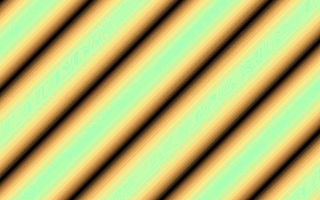# QB CULT MAGAZINEVol. 4 Iss. 1 - January 2004

## Plasmas Revealed

By Relsoft <vic_viperph@yahoo.com>

This time I'll try to explain what I know about generating Plasmas. I'm sure you've already seen different types of plasmas before. Basicaly, there are two ways to generate them:

1. Palette rotation
2. Functional( A lil Trig is needed)

Palette rotation is fast, very fast, but also static(not moving) except for the colors. Whereas Functional is a little slower but produces effects a *lot* better than the former. In fact the only limit to how good looking your plasmas would be, is your imagination. ;*) So I will teach you the second type of plasma generation, although you could combine both approaches to produce fantastic results. Also, using the functional approach would give the freedom to use it in games as it won't "touch" the other colors. I have made a 16 color plasma which I'll probably use in some of my games.

*Games that use this approach:

1. Chrono Trigger's warp effects
2. Bahamut Lagoon
3. Seiken Densetsu 3(Secret of Mana 2)
4. Final Fantasy series

#### The Palette:

How good-looking your plasmas depends on lots of factors, one of the more important factor is the palette. The color range should not be a problem as we could use as much and as less as we want. But it is very important to have a palette that wraps-around itself:#### The Sine function:

The Sine is part of those things Trig teachers call "circular functions". There are other circular functions but the SINE and COSINE functions are what is applied here. In fact I will only use the Sine function but you could use cosine once you learn how to generate the basic plasma.

One thing that makes the sine and cosine function great is the waves they generate when plotted on the Cartesian coordinate system. They produce easy to predict waveforms even if you introduce some nasty, out of this world displacement.Notice that both of the plotted coordinates does not go below -1 or above 1. This is the essence of our plasmas. In the above image, we applied the functions to the Y coordinate and the X coordinate as the parameter to the functions hence:

```y=sin(x)  and y=cos(x)
```

What if we apply the same functions to the color range of the palette? In this case 0 to 255 in screen 13(320*200*256).

#### Conversion:

```Radians=Degrees*PI/180
```

We will use this formula but with a "little" displacement(Imagination). ;*) It would still return -1 to 1.

Scale it by multiplying the color range you want affected. In this case, the whole 256 range. Q. But it would return a negative value? And I know there is no color -256. A. Yes, there is. Modular arithmetic. But that's for later. ;*)

```1. c% = (SIN(x% * PI / 270) * 256)
```+

```2. c%=(SIN(y% * PI / 45) * 32)
```=+

```3. c%=(SIN((y% + x%) * PI / 90) * 64)
```=That's it! You now have your basic plasma. To move the plasma is just to add an offset to the parameters so that every frame, the offset increments/decrements. Avra Kedavra!! Plasma movement. ;*) Darn Harry Potter book. Good read though (year 5) wink...wink... i.e:

```defint a-z
const PI=3.141593
cls
screen 13
do
F&=F&+1
for y=0 to 199
for x=0 to 319
C%=sin((x+y+f&)*PI/64)*255
next x
next y
loop until inkey\$<>""
```

Here's the commented code for all the stuff I ranted in this article: Note the use of Lookup tables to speed things up. ;*)

<PlasTutr.bas>

You could also try to use the polar coordinate system instead of the faster Cartesian coordinate system for a cooler plasma. ;*) Of course, you could also do this in textmode. ;*)

Here's are two examples:

<Plasdist.bas> <PlasText.Bas>

Before I forget, you could also do some nice pseudotranslucent plasmas. The trick to do it is to have 2 plasmas generated in one frame superimposed from each other at different frames. Here's an example:

<PlasTran.bas>

Let your imagination do the trick. Play with the functions, putting some out of this world parameters. You could also try to use the color itself as a parameter or even time. ;*)

Relsoft 2003
<vic_viperph@yahoo.com>
<http://rel.betterwebber.com>